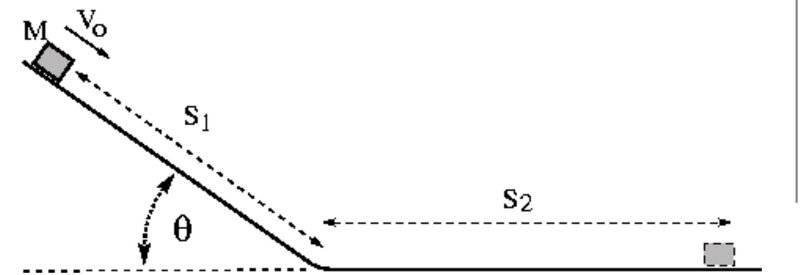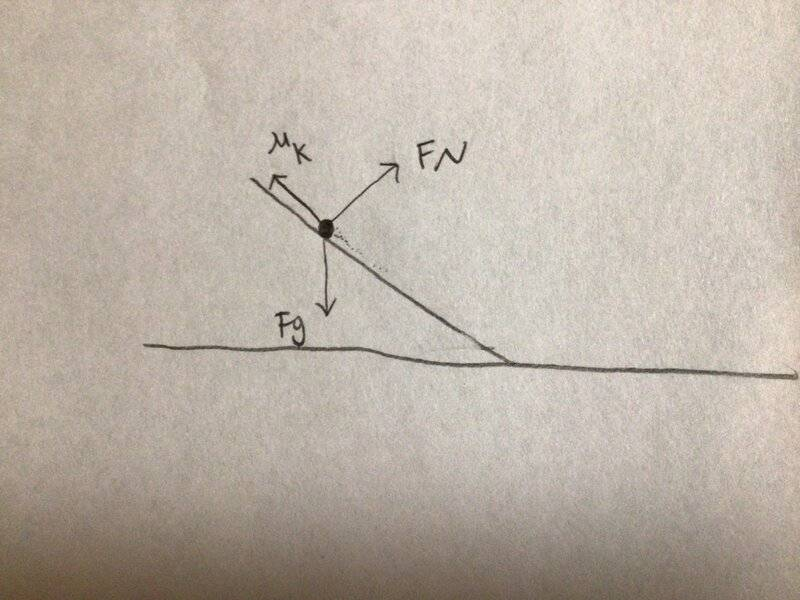# Physics homework help needed -- A mass sliding down a ramp with friction

• pilot12
In summary, the mass slides down the inclined part of the slide at a constant speed, and the coefficient of kinetic friction is equal to the normal force.

#### pilot12

Homework Statement
Trouble on this final problem of my physics homework
Relevant Equations
M g h + 1/2 mv^2 - (s1+s2)umg=0
h= 1.18sin(28.1) = .55579
When mass M is at the position shown, it is sliding down the inclined part of a slide at a speed of 2.19 m/s. The mass stops a distance S2 = 2.1 m along the level part of the slide. The distance S1 = 1.18 m and the angle θ = 28.10°. Calculate the coefficient of kinetic friction for the mass on the surface.
https://letu.loncapa.net/res/msu/physicslib/msuphysicslib/13_EnergyConservation/graphics/prob27a_MechEnWFriction.gifLast edited:
Hello @pilot12 ,##\qquad##!​

Here at PF we require an attempt at solution from you (see guidelines) before we are allowed to help.

Being confused is one thing, but being completely incapacitated is something else. What do you know that can help you here to get started ? (formulas, considerations, equations, etc)

##\ ##

Sorry I did not know that @BvU , I was trying to use

mgh + 1/2mv^2 - (s1+s2)u m g=0

h= 1.18sin(28.1) = .55579

pilot12 said:
Sorry I did not know that @BvU , I was trying to use

mgh + 1/2mv^2 - (s1+s2)u m g=0

h= 1.18sin(28.1) = .55579
Welcome to PF.

What you posted as the follow-up reply does not help. How are you gong to calculate the coefficient of friction to slow the mass in the specified distance?

Also, this might be a trick question. Is the problem saying that the mass slides down the incline with a constant speed? What would be significant about that?

@berkeman I believe it is saying it is sliding down at a constant speed. If it wasn’t, I think it would be an acceleration

pilot12 said:
@berkeman I believe it is saying it is sliding down at a constant speed. If it wasn’t, I think it would be an acceleration
Good. So what can you say about a value of kinetic friction on a ramp that allows constant velocity down the ramp?
What two forces are equal in that situation? Can you post a Free Body Diagram (FBD) of the mass while it is sliding down the ramp?

That the kinetic friction is equal to the Fnx? @berkeman##\mu_K## is not a force. ##\ \mu_K\times F_N\ ## is the friction force. What is ##F_N## in terms of ##F_g## and ##\theta## ?

@BvU I figured it out, the masses cancel out and then you can solve it. Appreciate your help!

DId you get a reasonable value for ##\mu## ?

pilot12 said:
@berkeman I believe it is saying it is sliding down at a constant speed. If it wasn’t, I think it would be an acceleration
No, I'm pretty sure it is not saying that. It may well be accelerating.
If you allow for that possibility, and just assume the coefficient is the same both on the slope and on the flat, there is enough information to solve it.
What value did you get?

•Delta2
It definitely accelerates on the slope, yes.

"When mass M is at the position shown, it is sliding down the inclined part of a slide at a speed of 2.19 m/s."
It is obvious from the text that it is sliding with that veleocity at that point and not for the whole length of the inclined plane. Otherwise, it should say "mass M is sliding down the inclined part of a slide at a speed of 2.19 m/s."

•Delta2
pilot12 said:
mgh + 1/2mv^2 - (s1+s2)u m g=0
The above equation looks like it is a correct application of the work energy theorem, however it is wrong. What is wrong is that the total work of friction is not ##(s_1+s_2)umg## because the normal force is not equal to ##mg## for the whole distance. It is equal to ##mg## only during the s2 distance.

Also we don't know if the friction coefficient is the same and equal to ##u## for the whole distance, we have to assume that otherwise it is not solvable by the data given.